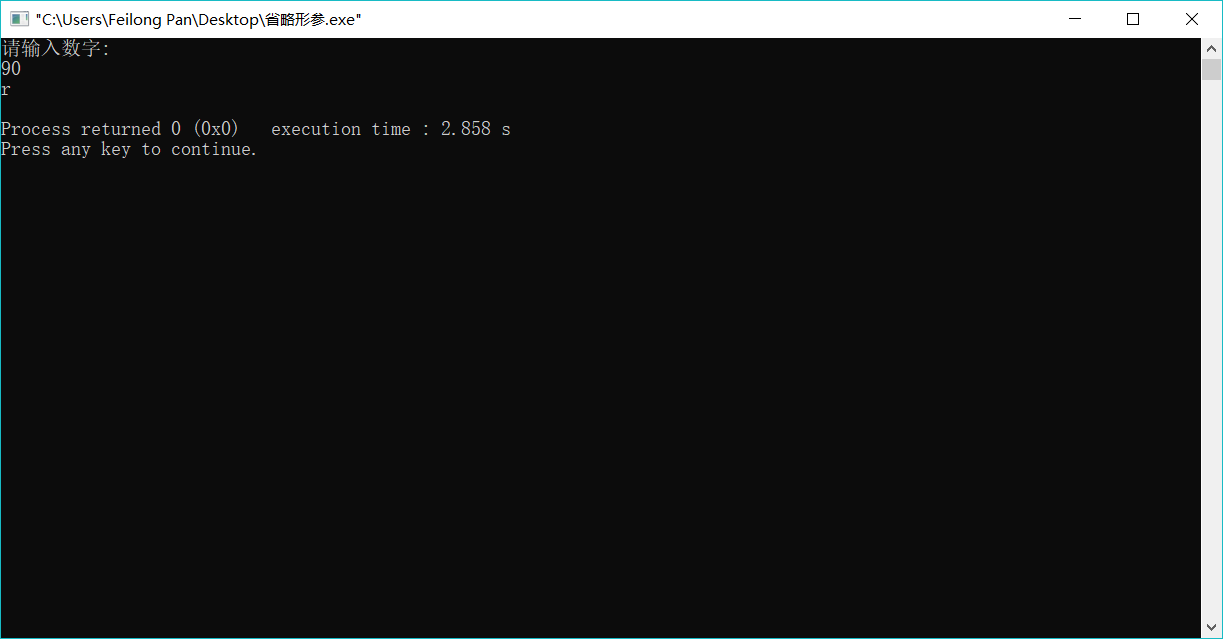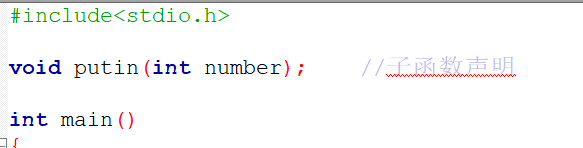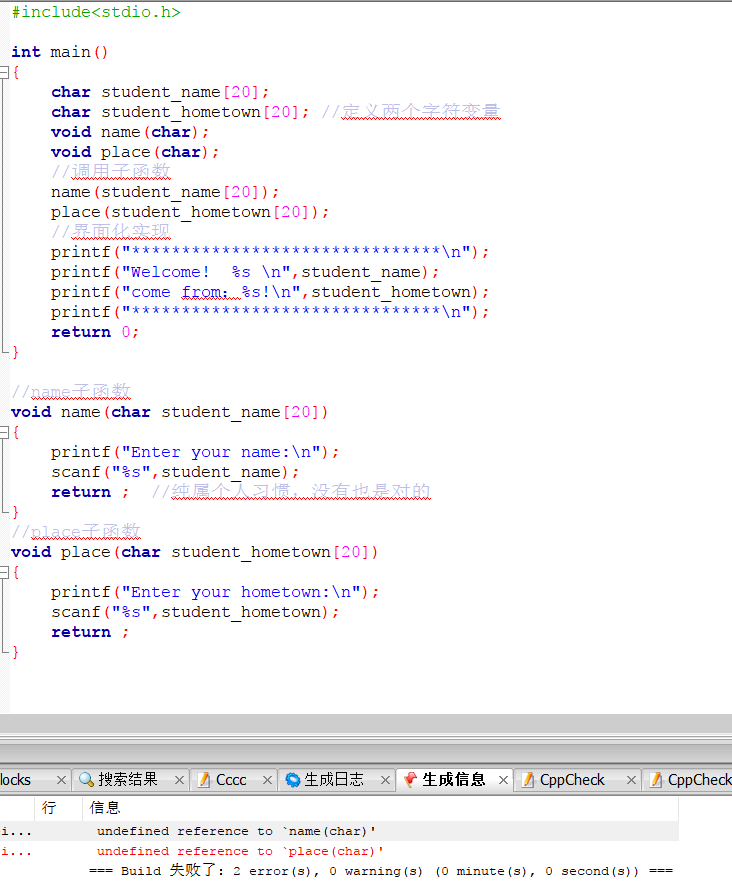• Matlab函数、子函数的定义方法
千次阅读
2021-04-20 04:26:20

Matlab函数、子函数的定义方法

除了最常使用的m文件函数，matlab还可以通过内联函数和匿名函数自定义函数。

1. m文件函数

以function关键字开头的m文件，并且通常函数名要与文件名相同。例如下面的内容保存为myfun2.m即是m文件函数，它用来求两数之和：

function f=myfun2(x,y)

f=x+y;

2. 子函数

matlab可以在m文件函数中定义一个函数和多个子函数，但是需要注意的是子函数只能由同一m文件中的函数调用。如下面的例子：

function [max,min]=mypfun(x) %主函数

n=length(x);

max=mysubfun1(x,n);

min=mysubfun2(x);

function r=mysubfun1(x,n) %子函数1

x1=sort(x);

r=x1(n);

function r=mysubfun2(x) %子函数2

x1=sort(x);

r=x1(1);

3. 内联函数

在函数文件内，m脚本和command窗口都可以用的一种定义函数的方式，但是要注意这种函数比较多的时候会占用比较多的内存空间。

如：

>> ci=inline('x.^2')

ci =

Inline function:

ci(x) = x.^2

4. 匿名函数

使用matlab函数句柄操作符@，可以定义指向matlab内置函数和用户自定义函数的函数句柄，函数句柄也可以像函数一样的使用。例如：

>>x=-pi:0.1:pi;

>> fh={@cos,@sin};

fh =

@cos @sin

>>plot(fh{2}(x))

matlab主程序调用子函数时变量代换问题

假如有一子函数

function fun1=(x)

a=[a1 a2 a3]

b=[b1 b2 b3]

p=polyfit(a,b,2)

y=poly2sym(p)

假如可得到结果

p1*x^2+p2*x+p3

现主程序需调用该函数，但自变量不是一维矢量x，而是二维x(j,i)或三维矢量x(j,i,k),

比如想得到：

p1*x(j,i)^2+p2*x(j,i)+p3

请高手指教如何在主程序中实现呢，十分感谢！

下面的例子可以参考一下，这样y=poly2sym(p)这句貌似就没什么用了.

如果你仅仅是想通过调用自己定义的函数得到一个具体的值，而不是想得到诸如p1*x^2+p2*x+p3这样的符号表达式，

那么我觉得就没必要用poly2sym.

另外，就算是想得到符号表达式，我觉得p1*x(j,i)^2+p2*x(j,i)+p3也是多余的。

因为我觉得，

p1*x^2+p2*x+p3中的x就可以代表二维x(j,i)或三维矢量x(j,i,k)的吧。

例子：

>> a=[1 2 3];

b=[1 2 3];

p=polyfit(a,b,2);

y=poly2sym(p)

y =

x

>> x0=[11,12];

>> y0=polyval(p,x0)

y0 =

11 12

更多相关内容
• matlab子函数调用方法说明-子函数调用.pdf 今天看了一下matlab子函数的调用，发现这个资料比较好，说的比较清楚，和大家共享一下。 子函数调用.pdf 子函数调用方法matlab
• 1. 高手指点一下Matlab的主函数和子函数的关系,怎么建立子函数主函数在结构上与其他函数没有一点区别，之所以称之为主函数，就是因为他在M文件上坐了第一把交椅，其他函数都排在他后面。按惯例他与M文件同名，在命令...

1. 高手指点一下Matlab的主函数和子函数的关系,怎么建立子函数

主函数在结构上与其他函数没有一点区别，之所以称之为主函数，就是因为他在M文件上坐了第一把交椅，其他函数都排在他后面。

按惯例他与M文件同名，在命令窗口或者其他函数中调用这个函数，都是引用M文件名。也有例外，当主函数与M文件不同名时，只能用文件名引用这个函数！M文件上的其他函数扮演着其的子函数角色。

一个M文件上排在主函数后面的都叫子函数，子函数的排列五规定顺序。子函数只能被同一个文件上的主函数或其他子函数调用。

子函数与子函数没有形式上的区别，每个子函数都有自己的函数定义行。几个子函数虽然在同一个文件上，但各有自己的变量，子函数之间不能相互存取别人的变量。

到那时，要是申明变量为全局变量，那个就另当别论。MATLAB的函数类型有以下几种：1、主函数2、子函数3、私有函数4、嵌套函数、5、重载函数6、匿名函数。

2. matlab主函数如何调用子函数

1、当你没有把你编辑好的子函数放入正确的文件夹时，不管你输入的程序是否有错，当你执行时，系统都会提示“没有定义”，这个时候就是没有子函数文件放到主函数库文件夹中，如图所示。

2、就算知道了，这个问题那么主函数库文件夹在什么地方找呢？首先，输入“edit conv”这个“conv”是我准备编辑的子函数的主函数名，每个人所需的主函数名都不一样，这里可以不要那么在意。然后就可以打开相应的主函数，这是可以看到主函数所在文件夹位置。

如图所示，※记住这个位置。 3、然后进行子函数的编辑，首先新建一个新的m文件，如图操作所示，然后在编辑框中输入所要的子函数，如图所示，例如我就是输入了convnem的函数，这是conv的子函数。

然后点击保存。 4、接下来，在刚刚第二步“conv”所在文件位置，将位置记录下来，将子函数的文件保存到主函数所在的文件夹即可，然后在重新的将你所需的程序(含有子函数的)输入并且执行，这时你会发现原来提示的错误没有了，那么这时调用自己添加的子函数成功了。

以后有相应的子函数都可以通过这样的方法进行添加子函数。 同一个脚本文件中若有多个函数，则分为主函数和子函数，每个M文件都要求有一个主函数，它最先出现在该m文件中，与文件同名。

主函数可以与该m文件外的其他函数相互作用，只要该文件所在目录属于matlab搜索路径；子函数subfunctions的执行范围：只能与该m文件中的其他函数相互作用，却不能被m文件之外的函数调用，子函数作为功能函数只是执行主函数的一部分工作。 Matlab- 主函数和子函数间接传递变量 在matlab一般可以通过函数的输入和输出接口来传递变量，但是有的时候，我们因为函数已经写好，并在比较多的脚本中被调用，此时再修改输入接口的工作量比较大，如果可以通过其它方式传递变量，就可以省去很多的工作量。

初步想了一下，可以通过两种形式实现： 1、在主函数中定义全局变量 但是这里需要注意的是全局变量y的定义必须包括在主函数的代码段中，如果放在主函数之外，或者定义在子函数中就无法被整个进程中的代码全局识别。 2、利用新的子函数定义来间接引入全局变量。

调用规则： 外层的嵌套函数的直接内层，例如varScope1可以调nestfun1，而nestfun1可以调用nestfun2，但varScope1不能调nestfun2； 有相同父函数的同层嵌套函数(本例不存在)； 内层的函数可以调用任何外层的函数，例如，nestfun2可以调用nestfun1和varScope1。 其他函数调用时，只能调最外面一层的函数(varScope1)，而不能调用调nestfun1和nestfun2。

变量作用域： 通常，函数的工作区只对其自身可见，其他函数不能访问。但对于嵌套函数而言，处于内层的函数可以访问外层函数的工作区(例如，nestfun2可以访问varScope1的局部变量x)，反之则不可以。

3. matlab中,怎么样在主函数里调用子函数

matlab可以在m文件函数中定义一个函数和多个子函数，但是需要注意的是子函数只能由同一m文件中的函数调用。如下面的例子：

function [max,min]=mypfun(x) %主函数

n=length(x);

max=mysubfun1(x,n);

min=mysubfun2(x);

function r=mysubfun1(x,n) %子函数1

x1=sort(x);

r=x1(n);

function r=mysubfun2(x) %子函数2

x1=sort(x);

r=x1(1);

4. matlab中主函数下面怎么添加子函数

function main

format long

i=145;

t=i*0.001;

x=zeros(2,1);

x(1,1)=35;

f=t*sin(x(1,1));

x(2,1)=Runge_Kutta(t,x(1,1))

%子函数内容为：

function x1=Runge_Kutta(x)

%Runge-Kutta积分方法解算

T=0.01;

%设周期是0.01秒，具体的计算中再修改

%f(x,t)=t*sin(x)； %这个语句肯定有问题，不起作用，所以注销掉

%先假设f(x,t)是一个这样的函数，具体函数在具体问题中再修改

x=x(1,1);

%x先假设一个值，到具体问题时再具体输入修改

K1=t*sin(x);

K2=(t+T/2)*sin(x+K1/2);

K3=(t+T/2)*sin(x+K2/2);

K4=(t+T)*sin(x+K3);

x1=x+(T/6)*(K1+2*K2+2*K3+K4);

%龙格库塔方法计算过程

以上计算的结果为

x =

35.000000000000000

34.999399730655441

展开全文• ## 子函数调用

千次阅读 2018-11-16 11:54:08
子函数调用 子函数 定义：能被其他程序调用，在实现某种功能后能自动返回到调用程序去的程序。其最后一条指令一定是返回指令，故能保证重新返回到调用它的程序中去。也可调用其他子程序，甚至可自身调用（如递归...
  						子函数调用

1. 子函数
定义：能被其他程序调用，在实现某种功能后能自动返回到调用程序去的程序。其最后一条指令一定是返回指令，故能保证重新返回到调用它的程序中去。也可调用其他子程序，甚至可自身调用（如递归）。
2. 函数的调用形式
函数调用的一般格式为：（在main函数中）
<函数名> （[<实际参数列表>]）；
3. 函数声明
函数声明的一般格式：
<返回类型><函数名> ([<形参类型1>][<形参1>]，[<形参类型2>][<形参2>]…);
例：
#include<stdio.h>

int main()
{
void putin(int);    //函数原型声明
int number;
printf("请输入数字:\n");
scanf("%d",&number);
putin(number);     //调用子函数putin()
return 0;
}

void putin(int number)
{
printf("%c\n",'number');  //将输入的数的ascll码输出
return ;
}


运行结果：注：
个人自己的理解：

1.在函数声明的时候，个人比较喜欢放到头文件的下面。声明时不是按照函数原型声明（省略形参），而是详细的都列出来，因为在用函数原型声明的时候很容易出错，倒不如直接全部声明。2.在函数调用的时候倒是没有什么不一样的，基本上就是这个模板.

最后在给一个你让我看的例子；

法一：

#include<stdio.h>
//声明子函数
void name(char student_name);
void place(char student_hometown);

int main()
{
char student_name;
char student_hometown; //定义两个字符变量
//调用子函数
name(student_name);
place(student_hometown);
//界面化实现
printf("*******************************\n");
printf("Welcome!  %s \n",student_name);
printf("come from：%s!\n",student_hometown);
printf("*******************************\n");
return 0;
}

//name子函数
void name(char student_name)
{
scanf("%s",student_name);
return ;  //纯属个人习惯，没有也是对的
}
//place子函数
void place(char student_hometown)
{
scanf("%s",student_hometown);
return ;
}


法二：

#include<stdio.h>
//声明子函数
/*
void name(char student_name);
void place(char student_hometown);
*/
//name子函数
void name(char student_name)
{
scanf("%s",student_name);
return ;  //纯属个人习惯，没有也是对的
}
//place子函数
void place(char student_hometown)
{
scanf("%s",student_hometown);
return ;
}

int main()
{
char student_name;
char student_hometown; //定义两个字符变量
//调用子函数
name(student_name);
place(student_hometown);
//界面化实现
printf("*******************************\n");
printf("Welcome!  %s \n",student_name);
printf("come from：%s!\n",student_hometown);
printf("*******************************\n");
return 0;
}



注：其实法二，并不算是一种方法。只是把所有的子函数放在了main函数的上边就不需要声明了。
遇到的一些问题：
1.尽量不要在声明的时候省略形参，其实这个我试了一下，报错了，也没调出来。
2.注意一下关于字符串的处理，值得深入研究字符串。
3.注释打一下，既是练打字，还能让自己更了解自己的代码，主要是能让别人读懂。

省略形参情况：所以以后尽量不要使用省略形参的情况！

展开全文函数调用
• 函数与子函数 函数语法结构 函数同其它高级语言一样，是MATLAB语言的重要组成部分，MATLAB除了内部函数外，还有各种工具箱中的函数，这些函数都以M文件给出，以便调用。 下面以一个例子来说明MATLAB函数的语法。...

## 函数与子函数

函数语法结构

• 函数同其它高级语言一样，是MATLAB语言的重要组成部分，MATLAB除了内部函数外，还有各种工具箱中的函数，这些函数都以M文件给出，以便调用。
• 下面以一个例子来说明MATLAB函数的语法。下面是文件名为mean.m的内容。
function y = mean(x,dim)
%MEAN   Average or mean value.
%   For vectors, MEAN(X) is the mean value of the elements in X. For
%   matrices, MEAN(X) is a row vector containing the mean value of
%   each column.  For N-D arrays, MEAN(X) is the mean value of the
%   elements along the first non-singleton dimension of X.
%
%   MEAN(X,DIM) takes the mean along the dimension DIM of X.
%
%   Example: If X = [0 1 2
%                    3 4 5]
%   then mean(X,1) is [1.5 2.5 3.5] and mean(X,2) is [1
%                                                     4]

%   Copyright 1984-2001 The MathWorks, Inc.
%   $Revision: 5.16$  $Date: 2001/04/15 12:01:26$

if nargin==1,
% Determine which dimension SUM will use
dim = min(find(size(x)~=1));
if isempty(dim), dim = 1; end
y = sum(x)/size(x,dim);
else
y = sum(x,dim)/size(x,dim);
end

• mean是MATLAB用于统计的一个函数。第一行为函数的声明，函数语法为：
function [返回参数列表]＝函数名(输入参数列表)


mean的返回参数为y，y可以用中括号括起来。输入参数为x和dim，

• nargin和nargout为MATLAB的内部变量，分别表示输入参数个数和输出参数个数，由于有些函数要进行参数个数检查或在一些函数实现时参数个数不确定，因此要借助这两个变量进行程序算法设计和控制。
• 百分号%用于注释，%后的字符串表示注释文本

函数编写示例

求任意两个自然数之间（包含两个自然数）所有自然数的和。

输入参数2各，输出参数1个，即返回和值。

函数名这里取为sum2，函数名要有一定意义，才便于记忆，而且不要于系统内部函数相同，可以通过“help 函数名”看一下改函数名是否为系统函数。

function r=sum2(n1,n2)
%sum2  求任意自然数n1和n2之间（含n1和n2）所有整数的和
if n2>=n1 ,
r =(n2-n1+1)*(n1+n2)/2;
else
r=(n1-n2+1)*(n1+n2)/2;
end
输入：
sum2(1,100)
ans =
5050


函数的参数

• 参数的传递规则

每个函数有自己专用的工作空间，函数内部变量与MATLAB工作空间的联系只通过输入参数与输出参数来建立。参数的传递属于值传递，即输入参数变量的值在函数内部改变了，而在调用改函数时没有作为返回参数，那么这个输入参数变量的并没有改变。
例如：

function r=test1(a)
%改变输入参数a的值
r=[];
a=999;
r=a;
运行：
a=100;test1(a);a
输出：
a =
100
说明a的值并没有改变，可以这样调用来改变a的值。
>> a=100;a=test1(a);a
输出：
a =
999


子函数
函数文件可以包含一个以上的函数，该文件中的第一个函数时主函数，后面定义的所有函数都是子函数，子函数只允许同一个文件中的函数及其它函数访问。函数文件名要与主函数名相同。

function s=mymainfun
num=input('输入一个正整数：')
sprintf('从1到整数%d的和为%d',num,mysum(num))
function s=mysum(num)
%求1到num所有整数相加之和
s=0;
for i=1:num,
s=s+i;
end


私有函数

私有函数指位于private目下的函数，它们只能被其上一层目录的函数访问，对于其它目录的函数是部可见的，这就允许私有函数可以与其它目录下的函数同名。

MATLAB在执行某个程序或函数时，如果它有私有目录，则先查找私有目录，在根据MATLAB的PATH查找其它目录下的函数。

如果建立了私有函数目录，则不要添加到MATLAB的查找路径当中。

建立内部函数
命令：

inline


语法：

inline(expr)  根据expr建立内部函数，函数自变量根据表达式自动搜索；
inline(expr, arg1, arg2, ...)  指定参数，arg1等参数是字符串参数
inline(expr, N)   参数为x,P1,P2,…,PN为


例子：

g = inline('t^2')
f = inline('xyz','x','y','z')
g = inline('x^P1+x^P2',2)
例子：运行如下
f = inline('x^2+y^3')

f =
Inline function:
f(x,y) = x^2+y^3

f(3,1)
ans =
10

展开全文• 一、.m内部的子函数： 在一个m文件中写多个函数,每个函数都以function开始,结束可以带end,也可以不带（这种情况下,出现另一个function或者到达文件结尾表明前一个function结束）. 案例1： 如果文件开头有 ...
• ## c语言学习+子函数

千次阅读 2019-10-25 13:46:42
0x01 子函数 实际参数 ———（数值）—- 形式参数 注意 c语言是传递值参并非变参。 并非 **引用传递 **–传递变参 （地址） 。 传值调用——传递地址 并非 数值。 例如: scanf ————值参做变参（送的地址&...
• import requests from lxml import etree import time import csv from urllib.request import ... # 定义函数抓取每页前30条商品信息 # 构造每一页的url变化 if __name__ == '__main__': for i in range(1,.
• 第三点：是(int a,int b) 这里面的a,b是参数的名字，int 表示的是函数参数的类型，这里的名字跟上面一样，你想写什么名字就写什么名字，这里面有两个，只要你在调用这个函数的时候里面的参数个数跟你的函数的参数...c语言
• c中将数组传递给子函数 如何在C中将数组传递给函数 (How to pass Array to a Function in C) Whenever we need to pass a list of elements as argument to any function in C language, it is prefered to do so ...
•c语言
• 题目：写几个函数 （1）输入十个员工的姓名和职工号； （2）按员工号由小到大顺序排序，姓名顺序也随之调整； （3）要求输入一个员工号，用折半查找找出该职工的姓名，从主函数输入要查找的职工号，输出职工的姓名；...c语言 选择排序法 折半查找法
• 如果您想要从函数返回一个一维数组，您必须声明一个返回指针的函数，如下： int *myFunction(void) { . . } 另外，C 不支持在函数外返回局部变量的地址，除非定义局部变量为 static 变量。 所以我们将数组a定义为...指针
• 主函数： （1）一个.m文件只有一个主函数，且正常情况下主函数函数名应该与文件名相同。 test.m文件： function [m,n] = test(x,y) %test function end ...子函数子函数子函数与主函数之间数...
• ## 父函数与子函数

千次阅读 2018-04-02 18:09:55
在父函数中调用子函数时，要如何使得父函数中的值也改变呢？我们以常见的数字交换问题来举例：如图所示：在子函数中打印结果正确，如图所示，在父函数中打印结果不正确。那么究竟是为什么不正确呢？原因在于，父函数...
• 如题 这是题目 下面是我编的代码 #include<stdio.h> int fun1(int a,int b){ int s=0; s=a+b; printf("%d",s); return 0; } int fun2(int a,int b){ int s=0; s=a-b; printf("%d",s);...int fun4(int ac语言
• #include int main() { ... 因为c函数参数传递机制是传值；数组名作为参数，本质是指针，故第一个实现了交换；而第二个直接传入值，相当于复制了一份数据，对原数据没有任何影响；（详见 函数；数组与指针 那两章；）
• 函数文件中的变量都是局部的，即一个函数文件中定义的变量不能被另一个函数文件或其它 M 文件使用 当函数调用完毕后，该函数文件中定义的所有局部变量都将被释放，即全部被清除 函数通过输入和输出参数与其它 M 文件...
• （1） 编制一个判定某数是否为素数的子函数prime （2） 键盘输入10个数据（这些数中有奇数、也有偶数）存入数组a中； （3） 输出a数组中的最大值和下标，以及输出其最小值和下标； （4） 通过调用子函数prime，找出...C++汇编 C语言
• double f1(int n, double a[], double x){} 子函数1 double Get_Run_Time(double (*f)(int, double *, double)){ 子函数2 (*f)(MAX, a, 1.1); } printf("Run time of f1: %.2e sec\n\n", Get_Run_Time...
• 1.先设置好当前文件的路径: 点击右上角带绿色箭头的小文件夹,设置当前程序所要存放的路径 点击右上角的新建,选择函数,在下图输入所创建的子函数,然后保存.  输入程序:   结果正确: ...
• 写一个头文件命名为max.h #ifndef MAX_H #define MAX_H void max(void); #endif 然后主函数的文件包含max.h头文件 #include <stdio.h>...子函数文件也要包含max.h #include <stdio.h&g...
• 如果子函数返回值是一个指针，在子函数中先用malloc定义了这个指针，然后return该指针。 子函数需要return一个内部分配的空间，那么释放就归调用者来free了，否则内部free再返回已经是无效空间。 ...
• 1.编写一个函数进行2个数值之间的加，减，乘，除运算，并将结果返回。 2. 输出下面的长方形图形要求可以指定行和列 3. 设某班学生5人信息分别为姓名、性别、成绩{“zhang”，‘m’，78}{“wang”，‘w’，66}{...c语言
• 利用子函数按照字符串大小对字符串数组进行排序` #include<stdio.h> #include<string.h> #define N 5 /***************************************** 函数功能：编写一子函数，实现按照字符串的大小将...数组 指针 排序
• 编写一个类似于sprintf的函数，与sprintf不同的是，参数多了一个等级参数，为unsigned char 型。 代码如下： #include <stdio.h> #include<stdarg.h> int my_sprintf(const unsigned char level,...
• 《Matlab子函数如何进行单步调试》 前言： 个人是Matlab小白，运用Matlab时，需要什么功能就去查度娘的那种。今天同事提供了一份Matlab写的算法程序，需要转换为FPGA硬件实现。于是遇到Matlab主程序调用的子函数，...参数问题...